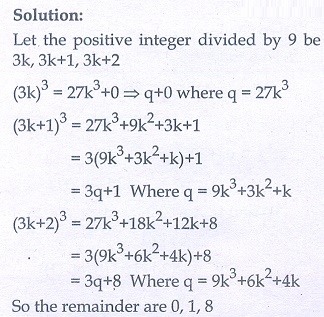# Maths - Online Test

Q1. If  n(A× B) = 6 and A = {1, 3} then n(B) is
Explaination / Solution: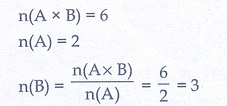Q2. Euclid’s division lemma states that for positive integers a and b, there exist unique integers q and r such that a = bq + r , where r must satisfy.
Explaination / Solution:
No Explaination.

Q3. A system of three linear equations in three variables is inconsistent if their planes
Explaination / Solution: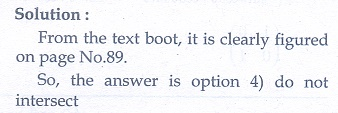Q4. If in triangles ABC and EDF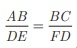then they will be similar, when
Explaination / Solution:Q5. The area of triangle formed by the points (−5,0) , (0,−5) and (5,0) is
Explaination / Solution: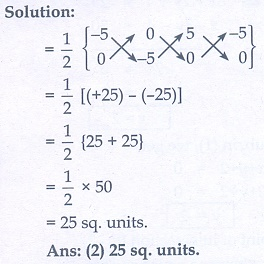Q6. The value of sin2θ +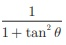is equal to
Explaination / Solution:
No Explaination.

Q7. The curved surface area of a right circular cone of height 15 cm and base diameter 16 cm is
Explaination / Solution: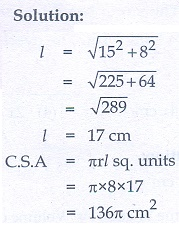Q8. Which of the following is not a measure of dispersion?
Explaination / Solution:
No Explaination.

Q9. A = {a,b, p},  B = {2, 3}, = {p,q,r,s} then n[(A C B] is
Explaination / Solution:Q10. Using Euclid’s division lemma, if the cube of any positive integer is divided by 9 then the possible remainders are
Explaination / Solution: